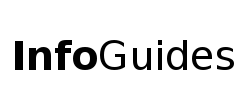# Learn R

Resources to learn and use the Open Source Statistical software R (R-Project)

#### Choose from the following:

Comparison (StackOverflow)

• ggplot2 – based on the book “The Grammar of Graphics” [MOST POPULAR]
• ggformula - uses formula notation and ggplot for ease of teaching
• lattice – implementation of Trellis graphics in R
• base r - use a series of functions to build your graph

#### Quick and Easy Exploratory Data Analysis

• ggquickeda - Provides a Point and Click Interface
• esquiesse - Helps build graphs with ggplot2
• GGally - Good for bivariate graphing with functions like ggpairs()

#### Interactive Graphics

• ggvis – make interactive graphics using ggplot2
• shiny – make interactive graphical applications using RStudio

## Network Analysis

Packages in R

• ggraph plots network graphs using the conventions and power of ggplot2.
• igraph is a collection of network analysis tools with the emphasis on efficiency, portability, and ease of use. igraph is open source and free, and can be used in R, Python, and C++.
• network manages relational data in R. Networks can be created with any combination of undirected/directed, valued/unvalued, dyadic/hyper, and single/multiple edges.
• networkD3 creates D3 JavaScript network, tree, dendrogram, aand Sankey graphs.
• tidygraph conceptualizes a network graph as two tidy data frames consisting of node and edge data.

Online courses and tutorials

Networkx is a Python package for the creation, manipulation, and study of the structure, dynamics, and functions of complex networks.

Networkx resources

NetworkX tutorials

Other Python tutorials